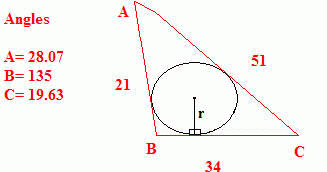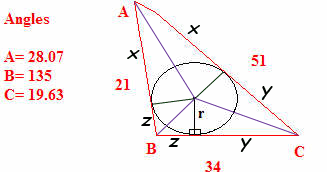SEARCH HOMEMath Central Quandaries & QueriesQuestion from Maria: Can you please help me, I need to find the radius (r) of a circle which is  inscribed inside an obtuse triangle ABC. (the circle touches all three sides of the triangle)I need to find r - the radius - which is starts on BC and goes up - up course the the radius creates two right angles on both sides of r. I can't thank you enough, MariaMaria, we have two responses for you:

Hi Maria.

Draw the radii to each of the three points of tangency and connect the vertices of the triangle to the center of the circle. Do you see that you have three pairs of congruent triangles? They are congruent because they are right triangles whose hypotenuses is shared and they have the same length of a leg (the radius).

Now there are three new variables to calculate (actually, just getting one of them is sufficient for your goal):Since these are congruent triangles, you know that angle C was divided exactly in half, so you know the measures of all the angles here. If you know the length y, then you can use the Tangent function to find the radius r. So now the problem is: what is y?

Actually, you can find that quickly by noticing that there are three equations and three variables:

x + z = 21
x + y = 51
y + z = 34.

Solve these simultaneous equations (using either the substitution or the elimination method) for y. Then use it in the Tangent function to find r.

Hope this helps,
Stephen La Rocque.

Hi Maria.

Stephen's answer overlooked a small problem: The angles cannot be very accurate -- they do not sum to 180 degrees.

Here is a formula in terms of the three sides:

If the sides have length a, b, c, we define the semiperimeter s to be half their sum, so s = (a+b+c)/2. Given this, the radius is given using the following:

r2 = (s - a)*(s - b)*(s - c) / s.

Take the square root of this expression to find r.

Prof. J. Chris Fisher.Math Central is supported by the University of Regina and The Pacific Institute for the Mathematical Sciences.